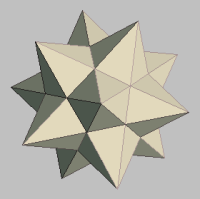MATHGOTSERVEDAdding and Subtracting Matrices
Learning Objective
On this page you will learn how to solve systems of two equations in two variables by:
1) Definition of Matrices
2) How to add and subtract matrices
Definition

Definition
A matrix can be defined as a rectangular array of objects into rows and columns. On this page we are going to be considering a rectangular array of numbers. These will be referred to as the elements of the matrix.

How to Add Matrices
1. First you must ensure that the two matrices have thesame dimension. That means that they should have thesame number of rows and columns. If their dimensions are equal then they could be added or subtracted. If not the result will be undefined.
2. Add corresponding elements of the two matrices.
3. The final matrix is your answer.
​​

mathgotserved.com
How to Add Matrices
VIDEO TUTORIALS
Interactive Practice
​Please wait for the test to load.....